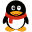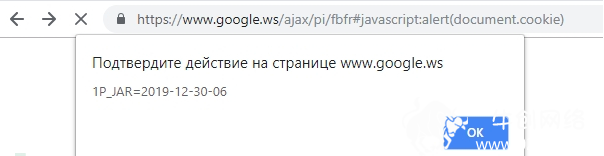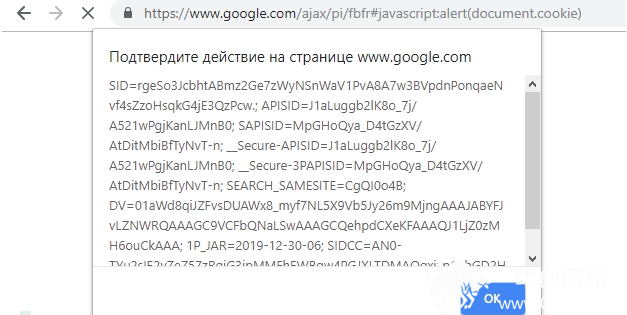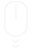7*24小时服务专线： 152-150-65-006 023-68263070## 漏洞公告团结互助，让我们共同进步！

Acunetix Vulnerability Scanner安全警告

首先，让我们看一下上个漏洞警告的HTTP响应内容，如下所示：

HTTP/1.1 200 OK

...

<!doctype html><div style="display:none"> <form method="post"> </form> <script nonce="+ao+4Egc+7YExl3qyyWMJg==">(function(){var a=window.document.forms,b=location.hash.substr(1);b||window.close();var c=b.split("&"),d=decodeURIComponent(c);a.action=d;for(var e=1;e<c.length;e++){var f=c[e].split("="),g=document.createElement("input");g.type="hidden";g.name=f;g.value=decodeURIComponent(f);a.appendChild(g)}a.submit();}).call(this);</script> </div>

分析表明，响应包括一个空的form格式和一些JS片段代码。为了获得更好的可读性，JS代码进行了以下重组：

(function() {

var a = window.document.forms,

b = location.hash.substr(1);

b || window.close();

var c = b.split("&"),

d = decodeURIComponent(c);

a.action = d;

for (var e = 1; e < c.length; e++) {

var f = c[e].split("="),

g = document.createElement("input");

g.type = "hidden";

g.name = f;

g.value = decodeURIComponent(f);

a.appendChild(g)

}

a.submit();

}).call(this);

接下来，我们将仔细分析上述JS代码。为此，我们添加一些注释以了解：

(function() {

// Function that is going to be auto-executed

}).call(this);

(function() {

// The variable “a” points to a form that is empty right now

var a = window.document.forms,

// The variable b is a location hash without the # character

b = location.hash.substr(1);

// If there is no b (no hash in the location URI), try to self-close

b || window.close();

// Split the location hash using the & character

var c = b.split("&"),

// And decode the first (zero) element

d = decodeURIComponent(c);

// The hash value becomes the action of the form

a.action = d;

// The below content is not important in the context of the issue

for (var e = 1; e < c.length; e++) {

var f = c[e].split("="),

g = document.createElement("input");

g.type = "hidden";

g.name = f;

g.value = decodeURIComponent(f);

a.appendChild(g)

}

// The form is auto-submitted

a.submit();

}).call(this);漏洞修复很简单，只需在上面的JS中添加一行代码即可0!= d.indexOf(“http”)&&window.close()，判断URL的location hash，即#后面的开始的字段以http开始，就能修复漏洞。

(function() {

var a = window.document.forms,

b = location.hash.substr(1);

b || window.close();

var c = b.split("&"),

d = decodeURIComponent(c);

// Only the below line needed to be changed

// to check if the location hash begins with http:

0 != d.indexOf("http") && window.close();

a.action = d;

for (var e = 1; e < c.length; e++) {

var f = c[e].split("="),

g = document.createElement("input");

g.type = "hidden";

g.name = f;

g.value = decodeURIComponent(f);

a.appendChild(g)

}

a.submit();

}).call(this);

### 热门资讯